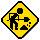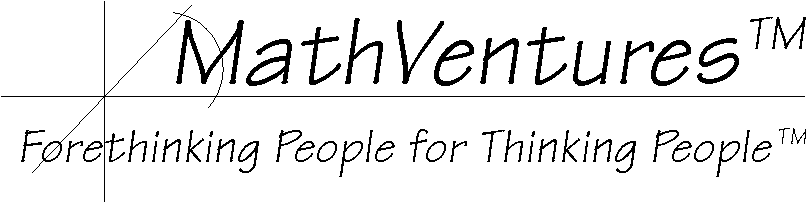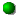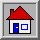Hard hat area, construction in progress# All-Around-Us Math# Look Around and Discover the MathSpending Time You do it every day. You barely give it a second thought. Yet, if you do it as you've been instructed, you will spend more than a day each year do it. What is it? Hint Solution The MathRatio and Proportion Machines There are a few ratio and proportion machines in your home. What are they? Hint Solution  The MathAdding Car Lane You are stuck in rush-hour traffic. All the highway lanes are packed. What if they add another lane, by how much will it reduce the traffic load on each existing lane? Hint Solution The Math

# HintsSpending Time You do it every day Puzzle Solution The MathRatio and Proportion Machines You use it every day, many times. It is up to you whether it is a ratio machine or a proportion machine. Sometimes both. Puzzle Solution  The MathAdding Car Lane Say there are now three lanes, what if they added a fourth one? Pazzle   Solution The MathSpending Time Brusing your teeth. Puzzle Hint The MathRatio and Proportion Machines The hot and cold water faucets in kitchens and bathrooms... Puzzle Hint  The MathAdding Car Lane By one fourth of the current traffic load. Pazzle   Hint The MathSpending Time If you brush your teeth twice daily for two minutes each time, each year you will spend 2 x 2 x 365 = 1,460 minutes. How many minutes are in one day or in 24 hours? 24 x 60 = 1,440 minutes. So brushing alone takes more than a day each year. Now add flossing...Puzzle Hint SolutionRatio and Proportion Machines The hot and cold water faucets in kitchens and bathrooms adjust the ratio of hot and cold water in the mix and that comes out of the spout. Once you like the temperature of the mix, adjusting the flow, you maintain the hot-to-cold ratio. In other words, you preserve the proportion. If you have a faucet with a single-lever control then in one direction it is a ratio machine and in the other it is a proportion machine.Puzzle Hint SolutionAdding Car Lane You might think that since 1 more lane is a third of the existing 3 lanes, the traffic load is reduced by 1/3. But you would be wrong. Let's use T to mark the total traffic load (for example, 3,000 cars per hour.) With 3 lanes the laod at each lane is T/3 or 4/12 of T. (In our example, each lane carries 1,000 cars per hour.) With 4 lanes the laod at each lane is T/4  or 3/12 of T. In other words, now each or the original 3 lane has 1/12 of T fewer cars. (In our example, each lane carries 750 cars per hour. For the fourth lane to have 750 cars, the load of each of the original 3 lanes was reduced by 250 cars, which is 1/4 of its original load. 1,000/250 = 1/4 So each of the 3 original lanes contributes 1/4 of its original load to the new lane.Pazzle   Hint Solution>